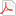Some integral transforms related to tetrationJmsNxnUltimate FellowPosts: 1,214 Threads: 126 Joined: Dec 2010 05/02/2013, 07:54 PM Hey everybody, I've just been working on some integral transforms related to tetration and was wondering if anyone could help me out. We first consider exponential space, which is the closure of the infinite linear span of exponential functions, so all functions of the form $e^{\lambda s}$ for $\lambda \in \mathbb{C}$ and linear combinations of them over complex numbers. We define the iterated derivative as $E: f(s) \to \frac{d^sf(t)}{dt^s}|_{t=0}$ $Ef(s) = \frac{1}{\Gamma(-s)} \int_0^\infty f(-y) y^{-s-1} dy$ which is just a rewritten form of Riemann Liouville's differintegral with lower limit negative infinity. It is clear one of its inverses is the taylor series so that $E^{-1} f = \sum_{n=0}^{\infty} f(n) \frac{s^n}{n!}$ However it also possesses another expression for its inverse: $E^{-1} f = \int_{-\infty}^{\infty} f(y) \frac{s^y}{\Gamma(y+1)} dy$ This took me a little while to prove but I've done it, and its in the following pdfExponential calculus.pdf (Size: 143.59 KB / Downloads: 731) It's still a rough paper and I'm planning to add a lot more. I'm still wondering about the conditions of convergence and some info about what functions this is legal for. For example, if we take a periodic function with period one and put it in the integral transform it is unlikely it equals the series transform. I.e: $\int_{-\infty}^{\infty} e^{2\pi y i } f(y) \frac{s^y}{\Gamma(y+1)} dy \neq \sum_{n=0}^{\infty} f(n) \frac{s^n}{n!}$ This has given me some great baffling, and I was wondering if someone might be able to explain why this happens. I've calculated the equivalence of the Taylor series transformation with the continuous transformation for some functions so I'm wondering how to classify them. Next I went on to define two transformations intimately related to tetration: $H f(s) = \int_{-\infty}^{\infty} f(y) (^y e)^s dy$ Defining $Tf(s) = f(s+1)$ we have $H [(^s e) f(s)] = T H f(s)$ and $E H f(s) = H T f(s)$ Defining $P f(s) = \sum_{n=0}^{\infty} f(n) (^n e)^s$ We see they satisfy the same equations, and so I am wondering if the two are equivalent up to constant for at least some extension of tetration. This is algebraically equivalent to how the taylor series equals the equivalent continuous transformation. For at least some functions. We also note that $E^n P f(s) |_{s=-\infty} = f(n)$ and so we are close to an inverse. My hypothesis is that the inverse of $H$ is $E^s f(t) |_{t=-\infty}$ But I have had little luck in finding such. An interesting set of functions are the two sets of eigenvectors of $E$ If we define $\psi, \phi$ as the following: $\psi(w,s) = \sum_{n=0}^{\infty} w^n (^n e)^s$ $\phi(w,s) = \int_{-\infty}^{\infty} w^y (^y e)^s dy$ They both satisfy $E \psi = w \psi$ and $E \phi = w \phi$ and they both converge for at least $\Re (s) < 1$ I'm wondering if it could be possible that they are equivalent. We also find that if we can analytically continue these functions to all $s \in \mathbb{C}$ then they are the sets of functions that satisfy: $\psi(w,s) = w \sum_{n=0}^\infty \psi(w,n) \frac{s^n}{n!}$ We can define, given the space of real exponential functions, the complex linear span of $e^{\lambda s} \,\,;\,\, \lambda \in \mathbb{R}$, an inner product which turns this in a Hilbert space: $(f,g) = \lim_{T \to \infty} \frac{1}{2T} \int_{-T}^T f(yi) \bar{g(yi)}dy$ This is very similar to the space of almost periodic functions insofar as it has the same inner product and orthonormal basis: $\{ e^{\lambda s}\,\,\lambda \in \mathbb{R}\}$ . We find a very curious result, that $(E^{-1} f, E^{-1} g) = (f,g)$ We could say that $E^{-1}$ is unitary but we run into some problems since by definition the kernel $ker(E) = \{0,1\}$ This is because $E$ is a closed operator, so: $E (1) = E \lim_{h\to 0} (1 + h)^s = \lim_{h\to 0}E (1 + h)^s = \lim_{h\to 0} \ln(1 + h) ^s = 0$ for all $\Re(s) > 0$ and since we are considering these functions globally, zero for all s. Of the most interest to me is the following relationship: $E (^w e)^s = (^{w-1} e)^s$ and so I was wondering if we could find a way of iterating $E$ we could arrive at a closed form expression for tetration, by example: $(1):\,\,\,\, E^{-y} e^s = (^{1+y} e)^s$ This reduces the problem of tetration to a problem in integral operators. So far I've had little luck with this problem. I thought expanding using the binomial theorem would work but that was to no avail. This does give an iterate but its solution does not satisfy the identity (1) I have a hunch that there is a way to iterate $E$ but it might involve using tetration rather than giving tetration, something that is slightly disappointing. « Next Oldest | Next Newest »

 Possibly Related Threads… Thread Author Replies Views Last Post Borel summation, Mellin Transforms, Parabolic iteration JmsNxn 5 1,668 09/10/2022, 03:12 PM Last Post: bo198214 A related discussion on interpolation: factorial and gamma-function Gottfried 9 20,754 07/10/2022, 06:23 AM Last Post: Gottfried Where is the proof of a generalized integral for integer heights? Chenjesu 2 5,907 03/03/2019, 08:55 AM Last Post: Chenjesu Not directly related to tetration, but still... Ansus 0 3,545 05/17/2017, 11:54 AM Last Post: Ansus 2 fixpoints related by power ? tommy1729 0 3,865 12/07/2016, 01:29 PM Last Post: tommy1729 Could be tetration if this integral converges JmsNxn 41 102,424 05/13/2014, 01:58 PM Last Post: JmsNxn [integral] How to integrate a fourier series ? tommy1729 1 5,981 05/04/2014, 03:19 PM Last Post: tommy1729 (draft) integral idea tommy1729 0 4,751 06/25/2011, 10:17 PM Last Post: tommy1729 Cauchy integral also for b< e^(1/e)? bo198214 14 28,253 04/24/2009, 05:29 PM Last Post: bo198214

Users browsing this thread: 1 Guest(s)